﻿ Why Does E=mc2 - Why Does E=mc²? (And Why Should We Care?) - Brian Cox, Jeffrey R. Forshaw ﻿

## Why Does E=mc²? (And Why Should We Care?) - Brian Cox, Jeffrey R. Forshaw (2009)

### Chapter 5. Why Does E=mc2?

In the last chapter we showed that merging space and time together into spacetime is a very good idea. Central to our whole investigation was the notion that distances in spacetime are invariant, which means that there is consensus throughout the universe as to the lengths of paths through spacetime. We might even regard it as a defining characteristic of spacetime. We were able to rediscover Einstein’s theory but only if we interpreted the cosmic speed limit c as the speed of light. We haven’t proved that c has anything to do with the speed of light yet, but we’ll dig much more deeply into the meaning of c in this chapter. In a sense, however, we have already begun to demystify the speed of light. Because the speed of light appears in E = mc2, it often seems as if light itself is important in the structure of the universe. But in the spacetime way of looking at things, light is not so special. In a subtle way, democracy is restored in the sense that everything hurtles through spacetime at the same speed, c, including you, planet Earth, the sun, and the distant galaxies. Light just happens to use up all of its spacetime speed quota on motion through space, and in so doing travels at the cosmic speed limit: The apparent special-ness of light is an artifact of our human tendency to think of time and space as different things. There is in fact a reason why light is forced to use up its quota in this way, and this is intimately related to our goal of understanding E = mc2. So, without further ado, let us continue on our quest.

E = mc2 is an equation. As we have been at some pains to emphasize, to a physicist equations are a very convenient and powerful shorthand for expressing relationships between objects. In the case of E = mc2the “objects” are energy (E), mass (m), and the speed of light (c). More generally, the objects living inside an equation could represent real material things, such as waves or electrons, or they could represent more abstract notions—such things as energy, mass, and distances in spacetime. As we have seen previously in this book, physicists are very demanding of their fundamental equations, for they insist that everyone in the universe should agree upon them. This is quite a demand—and at some time in the future we might discover that it is not possible to hold on to this ideal. Such a turn of events would be quite shocking for any modern physicist, since the idea has proved astonishingly fruitful since the birth of modern science in the seventeenth century.

As good scientists, however, we must always acknowledge that nature has no qualms about shocking us, and reality is what it is. For now, all we can say is that the dream remains intact. We explored this ideal of universal agreement earlier in the book and expressed it very simply: The laws of physics should be expressed using invariant quantities. All of the fundamental equations of physics that we know today achieve this by being written in such a way that they express relationships between objects in spacetime. What exactly does that mean? What is an object that lives in spacetime? Well, anything that exists presumably exists in spacetime, and so when we come to write down an equation—for example, one that describes how an object interacts with its environment—then we should find a way to express this mathematically using invariant quantities. Only then will everyone in the universe agree.

A good example might be to consider the length of a piece of string. Based on what we have learned, we can see that although the piece of string is a meaningful object, we should avoid writing down an equation that deals only with its length in space. Rather, we should be more ambitious and talk about its length in spacetime, for that is the spacetime way. Of course, for earthbound physicists it might be convenient to use equations that express relationships between lengths in space and other such things—certainly engineers find that way of going about things very useful. The correct way to view an equation that uses only lengths in space or the time measured by a clock is that it is a valid approximation if we are dealing with objects that move very slowly relative to the cosmic speed limit, which is usually (but not always) true for everyday engineering problems. An example we have already met where this is not true is a particle accelerator, where subatomic particles whiz around in circles at very close to the speed of light, and live longer as a result. If the effects of Einstein’s theory are not taken into account, particle accelerators simply stop working properly. Fundamental physics is all about the quest for fundamental equations, and that means working only with mathematical representations of objects that have a universal meaning in spacetime. The old view of space and time as distinct leads to a way of viewing the world that is something akin to trying to watch a stage play by looking only at the shadows cast by the spotlights onto the stage. The real business involves three-dimensional actors moving around and the shadows capture a two-dimensional projection of the play. With the arrival of the concept of spacetime, we are finally able to lift our eyes from the shadows.

All of this talk of objects in spacetime may sound rather abstract but there is a point to it. So far we have met one “mathematical representation of an object that has a universal meaning in spacetime”—the spacetime distance between two events. There are others.

Before we grapple with a new type of spacetime object we shall take one step back and introduce its analogue in the three dimensions of our everyday experience. It should come as no surprise (especially having read this far) that any reasonable attempt to describe the natural world exploits the notion of the distance between two points. Now, a distance is a special type of object—one that is characterized by a single number. For example, the distance from Manchester to London is 184 miles and the distance from the soles of your feet to the top of your head (more usually referred to as your height) is, at a guess, around 175 centimeters. The word following the number (cm or miles) just explains how we’re doing the counting but in both cases a single number suffices. The distance from Manchester to London provides some useful information—enough to know how much fuel to put in your car, for example, but not quite enough to make the journey. Without a map we might well head off in the wrong direction and end up in Norwich.

A slightly surreal and very impractical solution to that problem would be to construct a giant arrow whose length is 184 miles. We could place one end of the arrow in Manchester and the tip could sit in London. Arrows are useful objects when physicists set about the business of describing the world: They capture simultaneously the idea that something can have a size and also a direction. Obviously our giant Manchester-London arrow makes sense only once it is placed in a particular orientation; otherwise we might still end up in Norwich. That is what we mean when we say that the arrow has both size and direction. The arrows used by weather forecasters to illustrate how the wind blows provide another example of how arrows can help us describe the world. The swirling arrows capture the essence of the flow of the wind, telling us in which direction it blows at any particular point on the map as well as the wind speed: The bigger the arrow, the stronger the wind. Physicists call objects that are represented by arrows vectors. The wind speed as demonstrated on the weather map and the giant Manchester-London arrow are vectors in two dimensions, needing only two numbers for their description. For example, we might say that the wind is blowing at 40 miles per hour in a southeasterly direction. By showing us arrows in only two dimensions, the weather forecasters are not giving us the whole story—they are not telling us if the air is moving upward or downward and by what degree, but that isn’t something we are usually very interested in.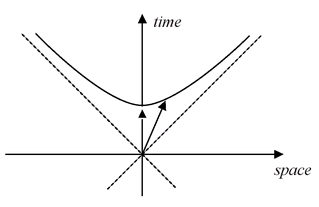FIGURE 9

There can also be vectors in three or more dimensions. If we began our journey from Manchester to London in one of the old villages in the Pennine Hills north of Manchester, we would have to point our arrow slightly downward since London sits on the banks of the River Thames at sea level. Vectors living in the three dimensions of everyday space are described by three numbers. By now, you might have guessed that vectors can also exist in spacetime, and these will be described by four numbers.

We are now about to reveal the two remaining pieces on the road to E = mc2. The first piece should come as no surprise—we are only ever going to be interested in vectors in the four dimensions of spacetime. That is easy to say but a weird concept: Just as a vector can point “north,” we now have the notion of a vector that points “in the time direction.” As is the norm when we talk about spacetime, this is not something we can picture in our mind’s eye, but that is our problem, not nature’s. The spacetime landscape analogy of the last chapter might help you build a mental picture (at least of a simplified spacetime with only one dimension of space). Four-dimensional vectors will be characterized by four numbers. The archetypal vector is the one that connects two points in spacetime. Two examples are illustrated in Figure 9. That one of the vectors in Figure 9 points exactly in the time direction and that both just happen to start out from the same place is only for our convenience. Generally speaking, you should think of any two points in spacetime with an arrow joining them. Vectors like these are not entirely abstract things. Your going to bed at 10 p.m. and subsequent awakening at 8 a.m. defines an arrow linking two events in spacetime; it is “10 hours multiplied by c long” and it points entirely in the time direction. Moreover, we have actually been using vectors in spacetime throughout the book but haven’t used the terminology before. For example, we met a very important vector in our discussion of the intrepid motorcyclist, journeying over the undulating landscape of spacetime with his throttle stuck. We worked out that the motorcyclist always travels at a speed c through spacetime, and the only choice he can make is the direction in which he points his motorcycle (although he doesn’t even have complete freedom of direction, because he is restricted to staying within a bearing of 45 degrees of north). We can represent his motion with a vector of fixed length c, which points in the direction in which he is traveling over the spacetime landscape. This vector has a name. It is called the spacetime velocity vector. To use the correct terminology, we would say that the velocity vector always has length c and is restricted to point within the future lightcone. The lightcone is a fancy name for the area contained within the two 45-degree lines that are so important in protecting causality. We can completely describe any vector in spacetime by specifying how much of it points in the time direction and how much of it points in the space direction.

By now, we are familiar with the statement that the distances in time and space between events are measured differently by observers moving at different speeds relative to each other, but they must change in such a way that the spacetime distance always remains the same. Because of the strange Minkowski geometry, this means that the tip of the vector can move around on a hyperbola that lies in the future lightcone. To be absolutely concrete, if the two events are “going to bed at 10 p.m.” and “waking up at 8 a.m.,” then an observer in the bed concludes that the spacetime distance vector points up his time axis, as illustrated in Figure 9, and its length is simply the time elapsed on his watch (10 hours) multiplied by c. Someone flying past at high speed would be free to interpret the person in bed as doing the moving. She would then have to add in a bit of space movement as well when she viewed the person in bed, and that moves the tip of the vector off her time axis. Because the arrow’s length cannot change, it must stay on the hyperbola. The second, tilted arrow in Figure 9 illustrates the point. As you can see, the amount of the vector pointing in the time direction has increased and this means that the fast-moving observer concludes that more time passes between the two events (i.e., more than 10 hours elapses on her watch). This is yet another way to picture the strange effect of time dilation.

So much, for now at least, for vectors (we will need the velocity spacetime vector again in a moment). The next few paragraphs relate to the second crucial piece of the E = mc2 jigsaw. Imagine you are a physicist trying to figure out how the universe works. You are comfortable with the idea of vectors and on occasion you have written down mathematical equations that contain them. Now suppose that someone, perhaps a colleague, tells you there is a very special vector, one that has the property that it never changes, no matter what happens to that part of the universe to which it corresponds. Your first reaction might be to express disinterest—if nothing changes then it is hardly likely to be capturing the essence of the matter at hand. Your interest would probably perk up if your colleague told you that the single, special vector is built up by adding together a whole bunch of other vectors, each associated with a different part of the thing you are trying to understand. The various parts of the thing can jiggle around and, as they do so, each of the individual vectors can change, but always in such a way that the sum total of all the vectors adds up to the same unchanging special vector. Incidentally, adding vectors together is easy, and we shall return to it in a moment.

To illustrate just how useful this idea of unchanging vectors can be, let’s think about a very simple task. We want to understand what happens when two billiard balls collide head-on. An example from billiards hardly sounds of earth-shattering significance but physicists quite often pick rather mundane examples like this, not because they can only study such simple phenomena or because they love billiards, but rather because concepts are often easiest to grasp first in simpler examples. Back to billiards: Your colleague explains that you should associate a vector with each ball. The vector should point in the direction of the ball’s motion. The claim is that by adding together the two vectors (one for each ball) we can obtain the special unchanging vector. That means that whatever happens in the collision, we can be sure that the two vectors associated with the balls after the collision will combine to make precisely the same vector as that obtained from the two balls before the collision. This is potentially a very valuable insight. The existence of the special vector severely limits the possible outcomes of the collision. We would be particularly impressed by our colleague’s claim that the “conservation of these vectors” works for every system of things in the whole universe, from colliding billiard balls to the explosion of a star. It will probably come as no surprise to know that physicists don’t go around referring to these as special vectors. Rather they speak of the momentum vector and the conservation of vectors is more commonly known as the conservation of momentum.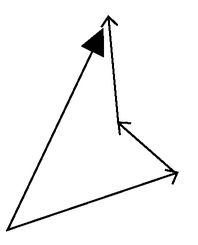FIGURE 10

We have left a couple of points hanging: Just how long are the momentum arrows and exactly how are we to add them together? Adding them together is not hard; the rule is to place all of the arrows that we want to add together end-to-end. The net effect is to define an arrow that links the start of the first arrow in the chain to the tip of the last arrow. Figure 10 shows how it is done for three randomly chosen arrows. The big arrow is the sum of the little ones. The length of a momentum vector is something we can ascertain from experiments, and historically this is how it was arrived at. The concept itself dates back over a thousand years, simply because it is useful. In a crude sense, it expresses the difference between being hit by a tennis ball or an express train when both are traveling at 60 miles per hour. As we have discussed, it is closely related to the speed and, as the previous example illustrates all too vividly, it should also be related to mass. Pre-Einstein, a momentum vector has length that is simply the product of mass and speed. As we have already said, it points in the direction of motion. As an aside, the modern view of momentum as a quantity that is conserved relates to the work of Emmy Noether, as we discussed earlier. Then we learned of the deep connection between the law of conservation of momentum and translational invariance in space. In symbols, the size of the momentum of a particle of mass m moving with a speed υ can be expressed as p = , where p is the commonly used symbol for momentum.

Up until now we have not really talked about what mass actually is, so before we proceed we ought to be a little more precise. An intuitive idea of mass might be that it is a measure of the amount of stuff something contains. Two bags of sugar have a mass twice that of one bag, and so on. Should we so desire, we could measure all masses in terms of the mass of a standard bag of sugar, using an old-fashioned set of balancing scales. This is how groceries used to be sold in shops. If you wanted to buy 1 kilogram of potatoes, you could balance the potatoes on a pair of scales against a kilogram bag of sugar, and everyone would accept that you had bought the right amount of potatoes.

Of course, “stuff ” comes in lots of different types, so “amount of stuff ” is horribly imprecise. Here is a better definition: We can measure mass by measuring weight. That is, heavier things have more mass. Is it that simple? Well, yes and no. Here on Earth, we can determine the mass of something by weighing it, and that is what everyday bathroom scales do. Everyone is familiar with the idea that we “weigh” in kilograms and grams (or pounds and ounces). Scientists would not agree with that. The confusion arises because mass and weight are proportional to each other if you measure them close to the surface of the earth. You might like to ponder what would happen if you took your bathroom scales to the moon. You would in fact weigh just over six times less than you do on Earth. You really do weigh less on the moon, but your mass has not changed. What has changed is the exchange rate between mass and weight, although twice the mass will have twice the weight wherever it is measured (we say that weight is proportional to mass).

Another way to define mass comes from noticing that more massive things take more pushing to get them moving. This feature of nature was expressed mathematically in the second most famous equation in physics (after E = mc2, of course): F = ma, first published in 1687 by Isaac Newton in his Principia Mathematica. Newton’s law simply says that if you push something with a force F, that thing starts to accelerate with an acceleration a. The m stands for mass, and you can therefore work out how massive something is experimentally by measuring how much force you have to apply to it to cause a given acceleration. This is as good a definition as any, so we’ll stick with it for now. Although if you have a critical mind you might be worrying as to how exactly we should define “force.” That is a good point but we won’t go into it. Instead we will assume that we know how to measure the amount of push or pull, a.k.a. force.

That was a fairly extensive detour, and while we haven’t really said what mass is at a deep level, we’ve given the “school textbook” version. A deeper view as to the very origin of mass will be the subject of Chapter 7, but for now it is presumed to “just be there”—an innate property of things. What is important here is that we are going to assume that mass is an intrinsic property of an object. That is, there should be a quantity in spacetime that everyone agrees upon called mass. This should therefore be one of our invariant quantities. We haven’t advanced any argument to convince the reader that this quantity necessarily should be the same as the mass in Newton’s equation, but as with many of our assumptions, the validity or otherwise will be tested when we have derived the consequences. We will now return to billiards.

If the two balls collide head-on, and they have the same mass and the same speed, then their momentum vectors are equal in length but point in opposite directions. Add them together and the two cancel each other entirely. After the collision, the law of momentum conservation predicts that whatever the particles will be doing, they must come off with equal speeds and in opposite directions. If this were not the case, then the net momentum afterward could not possibly cancel out. The law of momentum conservation is, as we said, not confined to billiard balls. It works everywhere in the universe, and that is why it is so very important. The recoil of a cannon after it shoots a cannonball or the way in which an explosion sprays particles in every direction are both in accord with momentum conservation. Actually, the case of the cannonball is worth a little more of our attention.

Before the cannon is fired, there is no net momentum and the cannonball is sitting at rest inside the barrel of the cannon, which is itself standing still on top of a castle. When the cannon is fired, the cannonball shoots out at high speed, while the cannon itself recoils a bit but stays pretty much where it began, fortunately for the soldiers in the castle who fired it. The cannonball’s momentum is specified by its momentum vector, which is an arrow whose length is equal to the mass of the ball multiplied by its speed and whose direction points away from the cannon along the direction of flight as it emerges from the barrel. Momentum conservation tells us that the cannon itself must recoil with a momentum arrow that is exactly equal in length but opposite in direction to the arrow associated with the ball. But since the cannon is much heavier than the ball, the cannon recoils with much less speed. The heavier the cannon, the slower it recoils. So, big and slow things can have the same momentum as small and fast ones. Of course, both the cannon and the ball slow down eventually (and lose momentum as a result), and the ball changes its momentum because it is acted on by gravity. However, this does not mean that momentum conservation has gone wrong. If we could take account of the momentum taken by the air molecules that collide with the ball and the molecules inside the bearings of the cannon, and the fact that the momentum of the earth itself changes slightly as it interacts with the ball through gravity, then we would find that the total momentum of everything would be conserved. Physicists usually cannot keep track of where all of the momentum is going when things like friction and air resistance are present, and as a result the law of momentum conservation is usually applied only when external influences are not important. It is a slight weakening of the scope of the law, but it ought not to detract from its significance as a fundamental law of physics. That said, let’s see if we can finish our game of billiards, which is dragging on somewhat.

To simplify matters, imagine that frictional forces are completely removed so that all we have to think about are the colliding billiard balls. Our newfound law of momentum conservation is very valuable but it isn’t a panacea. It isn’t in fact possible for us to figure out the speed of the billiard balls after their collision knowing only that momentum is conserved and the masses and velocities of the balls before the collision. To be able to work this out, we need to make use of another very important conservation law.

We have introduced the ideas that moving things can be described by a momentum vector and that the sum of all momentum vectors remains constant for all time. Momentum is interesting to physicists precisely because it is conserved. It is important to be clear on this fact. If you don’t like the word “momentum,” then you could do much worse than to speak of “the arrow that is conserved.” Conserved quantities are, as we are beginning to discover, rather numerous and exceedingly useful in physics. Generally speaking, the more conservation laws you have at your disposal when tackling a problem, the easier it will be to find a solution. Of all the conservation laws, one stands out more than any other, because of its profound usefulness. Engineers, physicists and chemists uncovered it very slowly during the course of the seventeenth, eighteenth, and nineteenth centuries. We are speaking of the law of conservation of energy.

In the first instance, energy is an easier concept to grasp than momentum. Like momentum, things can have energy but, unlike momentum, energy has no direction. In that respect it is more like temperature, in that a single number will suffice to specify it. But what is “energy”? How do we define it? What is it measuring? Momentum was easy in that regard: An arrow points in the direction of motion and is of a length equal to the product of the mass and the speed. Energy is less easy to pin down, because it can come in many different guises, but the bottom line is clear enough: Whatever happens, the sum total of all the energy in any process should remain unchanged regardless of how things might be changing. Again, Noether gave us the deep explanation. The conservation of energy arises because the laws of physics remain unchanged with time. That statement does not mean that things do not happen, which would obviously be silly. Instead it means that if Maxwell’s equations hold true today, then they ought also to hold true tomorrow. You can replace “Maxwell’s equations” with any fundamental law of physics—Einstein’s postulates, for example.

That said, and as with the conservation of momentum, the conservation of energy was first discovered experimentally. The story of its discovery is a meander though the history of the Industrial Revolution. It sprang from the work of many a practical experimenter who came across an immense variety of mechanical and chemical phenomena in pursuit of industrial Jerusalem. Men like the unfortunate Count Rumford of Bavaria (born Benjamin Thompson in Massachusetts in 1753), whose job it was to bore cannon for the Duke of Bavaria. While boring away, he noticed that the metal of the cannon and the drill bit got hot, and correctly surmised that the rotational motion of the drill was being converted into heat by friction. This is the opposite of what happens in a steam engine, in which heat gets converted into the rotary motion of the wheels of a train. It seemed natural to associate some common quantity with heat and rotational motion, since these seemingly different things appear interchangeable. This quantity is energy. Rumford has been termed unfortunate because he married the widow of another great scientist, Antoine Lavoisier, after Lavoisier lost his head to the guillotine in the French Revolution, in the mistaken belief that she would do for him as she had for Lavoisier and dutifully take notes and obey him as a good eighteenth-century wife should. It turned out that she had been submissive only under the duress of Lavoisier’s iron will, and in his rather wonderful book The Quest for Absolute Zero, Kurt Mendelssohn described her as leading him “a hell of a life” (the book was written in 1966, hence the quaint turn of phrase). The key point is that energy is always conserved, and it is because it is conserved that it is interesting.

Ask someone on the street to explain what energy is and you’ll get either a sensible answer or a pile of steaming New Age nonsense. There is such a wide spectrum of meanings in popular culture because “energy” is a word that is widely used. For the record, energy has a very precise definition indeed and it cannot be used to explain ley lines,5 crystal healing, life after death, or reincarnation. A more sensible person might answer that energy can be stored away, inside a battery waiting in suspension until someone “completes the circuit”; it could be a measure of the amount of motion, with faster objects having more energy than slower ones. Energy stored in the sea or in the wind provide particular examples of that. Or perhaps you would be told that hotter things contain more energy than colder ones. A giant flywheel inside a power station can store up energy, to be released onto the national electrical grid to meet the demands of an energy-hungry population, and energy can be liberated from inside an atomic nucleus to generate nuclear power. These are just some of the ways we might encounter energy in everyday life, and they can all be quantified by physicists and used to balance the books when it comes to making sure that the net effect of any process is such that the total energy remains unchanged.

To see energy conservation in action in a simple system, let us return to the colliding billiard balls for the final time. Before they hit each other, each ball has some energy due to its motion. Physicists call that type of energy kinetic energy. The Oxford English Dictionary defines the word “kinetic” to mean “due to or resulting from motion,” so the name is sensible. We previously assumed that the balls were traveling at equal speeds and had the same mass. They then collide and head out at equal speeds and in opposite directions. That much is dictated by momentum conservation. Closer inspection reveals that their outgoing speed is a little less than the speed before the impact. That is because some of the initial energy has been dissipated in the collision. The most apparent dissipation occurs with the emission of sound. As the balls collide, they agitate the molecules in the surrounding air, and this disturbance makes its way to our ears. So some of the initial energy leaks away, leaving less for the outgoing billiard balls. As far as our journey in this book is concerned, we don’t actually need to know how to quantify energy in all of its different guises, although the formula for kinetic energy will turn out to be useful later. To anyone who has a little experience in high school science, it will be indelibly imprinted deep within their psyche: kinetic energy =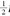. The main thing is to realize that energy can be quantified in a single number and, provided we are careful with the bookkeeping, the total energy in a system remains constant for all time.

Now let us get back to the point. We introduced momentum as an example of a quantity that is described by an arrow and, along with energy, its utility arises out of the fact that it is a conserved quantity. That all seems well and good but a huge dilemma is lurking in the shadows. Momentum is an arrow that lives only in the three dimensions of our everyday experiences. Generally speaking, a momentum arrow can point up or down or southeast or in any other direction in space. This is because things can and do fly around in any direction in space, and the momentum arrow captures the direction of motion. But the whole point of the last chapter was to expose our tendency to isolate space and time as a fallacy. We need arrows that point in the four dimensions of spacetime; otherwise, we’ll never be able to build fundamental equations that respect Einstein. To reiterate: Fundamental equations should be built out of objects that live in spacetime, not objects that live in space or in time separately because those types of object are subjective. Recall that neither the length of an object in space nor the time interval between two events are quantities whose values everyone will agree upon. That is what we mean when we say they are subjective. Likewise, momentum is an arrow that points somewhere only in space. That bias against time sows the seeds of its destruction. Does spacetime herald the breakdown of this most fundamental of laws in physics? It is true that our newly discovered structure of spacetime sows the seeds of destruction but it also indicates how we should proceed: We need to find an invariant quantity to replace the old three-dimensional momentum. This is a key point in our narrative: Such a thing does exist.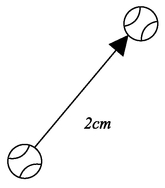FIGURE 11

Let’s take a closer look at the three-dimensional momentum vector. Figure 11 shows an arrow in space. It might represent the amount by which a ball moves as it rolls across a table.6 To be more precise, suppose that at midday the ball is at one end of the arrow, then 2 seconds later it is at the other end, the tip. If the ball moves 1 centimeter each second, then the arrow is 2 centimeters long. The momentum vector is easy to obtain. It is an arrow pointing in exactly the same direction as the arrow in Figure 11 except that its length is different. The length is equal to the speed of our ball (in this case 1 centimeter per second) multiplied by the mass of the ball, which we might suppose to be 10 grams. Physicists would say that the momentum vector of the ball has a length of 10 gram-centimeters per second (which they would abbreviate to something like 10 g cm/s). It is again going to be well worth our while to be a little bit more abstract and introduce placeholders rather than commit to any particular mass or speed. As ever, we certainly do not wish to transmogrify into the school mathematics teachers of our youth. But . . . if Δx is a placeholder for the length of the arrow, Δt is the time interval, and m is the mass of the ball (Δx = 2 centimeters, Δt = 2 seconds, and m = 10 grams in the example), then the momentum vector has a length equal to mΔxt. It is common in physics to use the Greek symbol Δ(pronounced “delta”) to represent “difference,” and in that spirit Δt stands for the difference in time or the time interval between two things, and Δx stands for the length of something, in this case the distance in space between the start and the end of our measurement of the ball’s position.

We have succeeded in constructing the momentum vector of a ball in three-dimensional space, although it is hardly the most exciting thing we have done. We’re now going to make the bold step of trying to build a momentum vector in spacetime, and we will do it in an entirely analogous way to the three-dimensional case. The only constraint is that we will use only objects that are universal in spacetime.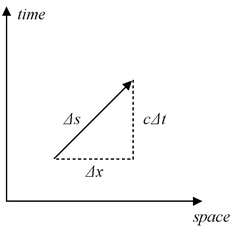FIGURE 12

Again we shall start with an arrow, this time pointing in four-dimensional spacetime, as illustrated in Figure 12. One end of the arrow specifies where our ball is at one instant and the other end specifies where it is some time later. The length of the arrow must be determined by Minkowski’s formula for the distance in spacetime, and it is therefore specified by (Δs2 = (cΔt)2 - (Δx)2. Remember that Δs is the only length that everyone in the universe can agree upon (something that most definitely cannot be said for Δx and Δt separately), and as such it is the distance measurement we must use, taking the place of Δx in the three-dimensional definition of momentum. But what is to take the place of the time interval Δt? (Remember, we are trying to find a four-dimensional replacement for mΔxt). Here comes the crunch: We cannot use Δtbecause it is not a spacetime invariant. Not everyone agrees on time intervals, as we have emphasized again and again, and therefore we must not use time intervals in our quest for the four-dimensional momentum. What are our choices? By what could we possibly divide the length of the arrow by to determine the ball’s speed through spacetime?

We want to construct something that is an improvement over the old three-dimensional momentum. If we are dealing with objects moving around at speeds that are slow compared to the speed of light, then we should find that the new momentum is at least approximately equivalent to the old one. If that is to happen, we must divide the length of our arrow in spacetime Δs by some quantity that is of the same type as an interval in time. Otherwise the new four-dimensional momentum will be an entirely different beast from the old three-dimensional momentum. Intervals of time can be measured in seconds, so we would also like something that can be measured in seconds. Starting from our invariant spacetime quantities, the speed of light c and the distance Δs, there is only one viable combination: It is the number we obtain upon dividing the length of the arrow (Δs) by the speed c. In other words, if Δs is measured in meters, and the speed c is measured in meters per second, then Δs/c is measured in seconds. This must be the number we need to divide the length of our arrow by, since it is the only invariant thing we have at our disposal that is measured in the correct currency. So let us go ahead and divide Δs by the time Δs/c. The answer is simply c (for much the same reason that 1 divided by ½ is equal to 2). In other words, the four-dimensional analogue of the speed in our three-dimensional momentum formula is the universal speed limit c.

This all might feel rather familiar, and that is because it should be familiar. All we have done is to calculate the speed of an object (a ball in our example) in spacetime and found it to be c. We came to exactly the same conclusion in the previous chapter when we considered the motorcyclist moving over the spacetime landscape. From the perspective of this chapter, we have done rather more because we have also found a spacetime velocity vector that has the potential to be used in a new definition of four-dimensional momentum. The velocity of an object moving through spacetime always has length c and it points in the direction in spacetime in which the object travels.

To finish our construction of the new spacetime momentum arrow, all we need to do is multiply the spacetime velocity vector by the mass m. It follows that our proposed momentum arrow always has a length equal to mc and points in the direction of travel of the object in spacetime. At first glance this new momentum arrow is a little boring because its length in spacetime is always the same. It seems we are hardly off to a good start. But we should not be deterred. It remains to be seen whether the spacetime momentum vector that we have just constructed bears any relation to the old-fashioned three-dimensional momentum or, for that matter, whether it will be of any use to us in our new spacetime world.

To delve a little deeper, we will now take a look at the portions of our new spacetime momentum vector that point in the space and time directions separately. To do this bit of delving, we need a bit of absolutely unavoidable mathematics. We can only apologize to the nonmathematical reader and promise that we will go very slowly. Remember, it is always an option to skim over the equations in search of the punch line. The mathematics makes the argument more convincing but it is okay to read on without following the details. Similarly, we must also apologize to the reader familiar with mathematics for laboring the point. We have a saying in Manchester: “You can’t have your cake and eat it.” This saying is perhaps harder to understand than the mathematics.

Recall that we arrived at an expression for the length of the momentum vector in three-dimensional space, mΔxt. We have just argued that Δx should be replaced by Δs and Δt should be replaced by Δs/c to form the four-dimensional momentum vector, which has a seemingly rather uninteresting length of mc. Indulge us for one more paragraph, and let us write the replacement for Δt, i.e., Δs/c, in full. Δs/c is equal to √(cΔt)2 - (Δx2 /c. This is a bit of a mouthful, but a little mathematical manipulation allows us to write it in a simpler form, i.e., it can also be written as Δt/γ where γ = 1/ √1—υ2/c2. To obtain that, we have used the fact that υ = Δxt is the speed of the object. Now γ is none other than the quantity we met in Chapter 3 that quantifies the amount by which time slows down from the point of view of someone observing a clock fly past at speed.

We are actually nearly where we want to be. The whole point of that piece of mathematics is that it allows us to figure out by exactly how much the momentum vector points off in the space and time directions separately. First let’s recap how we dealt with the momentum vector in three-dimensional space. Figure 11 helped us picture this. The three-dimensional momentum vector points off in exactly the same direction as the arrow in Figure 11, because it points in the same direction that the ball is moving in. The only difference is that its length is changed because we need to multiply it by the mass of the ball and divide by the time interval. The situation is entirely analogous in the four-dimensional case. Now the momentum vector points off in the direction in spacetime in which the ball is moving, which is the direction of the arrow in Figure 12. Again, to get the momentum, we need to rescale the length of the arrow, but this time we are to multiply by the mass and divide by the invariant quantity Δs/c (which we showed in the last paragraph is equal to Δt/γ). If you look carefully at the arrow in Figure 12, you should be able to see that if we want to change the length by some amount while keeping it pointing in the same direction, then we must simply change the bit pointing in the x direction (Δx) and the bit pointing in the time direction (cΔt) by the same amount. So, the length of the part of the momentum vector that points in the space direction is simply Δxmultiplied by m and divided by Δt/γ, which can be written as γmΔxt. Remembering that υ = Δxt is the speed of the object through space, we have the answer: The part of the momentum spacetime vector that points in the space direction has a length equal to γmυ.

Now that really is interesting—the momentum vector in spacetime that we just constructed is not boring at all. If the speed υ of our object is much less than the speed of light c, then γ is very close to one. In that case, we regain the old-fashioned momentum, namely the product of the mass with the speed p = mυ. This is very encouraging—we should press on. In fact, we have done much more than translate the old-fashioned momentum into the new four-dimensional framework. For one thing, we have what is presumably a more accurate formula since γ is only ever exactly one when the speed is zero.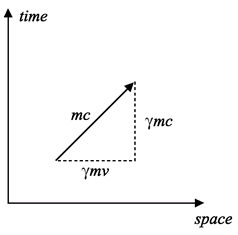FIGURE 13

More interesting than the fact that we have modified p =  is what happens when we consider that part of the momentum vector that points off in the time direction. After all of the hard work we have been investing, it is not hard for us to compute it, and Figure 13 shows the answer. That part of the new momentum vector that points off in the time direction has a length equal to cΔt multiplied by m and divided by Δt/γ again, which is γmc.

Remember, momentum is interesting to us because it is conserved. Our goal has been to find a new, four-dimensional momentum that will be conserved in spacetime. We can imagine a bunch of momentum vectors in spacetime, all pointing off in different directions. They might, for example, represent the momenta of some particles that are about to collide. After the collision, there will be a new set of momentum vectors, pointing in different directions. But the law of momentum conservation tells us that the sum total of all the new arrows must be exactly the same as the sum total of the original arrows. This in turn means that the sum total of the portions of each of the arrows pointing in the space direction must be conserved, as should the sum of the portions pointing in the time direction. So if we tally up the values of γmυ for each particle, then the grand total before the collision should be the same as the value afterward. Likewise for the time portions, but this time it is the sum total of the γmc values that is conserved. We appear to have two new laws of physics: γmυ and γmc are conserved quantities. But what do these two particular things correspond to? At first sight, there is nothing much to get excited about. If speeds are small, then γ is very close to 1 and γmυ simply becomes . We have therefore regained the old-fashioned law for momentum conservation. This is reassuring since we hoped that we would arrive at something that Victorian physicists would recognize. Brunel and the other great engineers of the nineteenth century certainly managed just fine without spacetime, so our new definition of momentum really had to give rise to almost the same answers as it did during the Industrial Revolution, provided things are not whizzing around at too close to the speed of light. After all, the Clifton Suspension Bridge did not suddenly cease to remain suspended when Einstein came up with relativity.

What can we say about the conservation of γmc? Since c is a universal constant upon which everyone always agrees, then the conservation of γmc is tantamount to saying that mass is conserved. That doesn’t seem a big surprise and it is in accord with our intuition, although it is rather interesting that it has popped out as if from nowhere. For example, it seems to say that after burning coal in a fire, the mass of the ashes afterward (plus the mass of any matter that went up the chimney) should be equal to the mass of the coal before the fire was lit. The fact that γ isn’t exactly one hardly seems to matter, and we might be tempted to move on, satisfied that we have already achieved a great deal. We have defined momentum in such a way that it is a meaningful quantity in spacetime and as a result we have derived (usually tiny) corrections to the nineteenth-century definition of momentum while simultaneously deriving the law of conservation of mass. What more could we hope for?

It has taken us a long time to reach this point, but there is a sting in the tail of this narrative. We are going to take a closer look at that part of the momentum vector that points off in the time direction, and in so doing we will, almost miraculously, uncover Einstein’s most famous formula. The finale is within sight. Thales of Miletus is reclining in his bath, preparing for the ultimate enchantment. In following the book up to this point, you may well be juggling a lot of mental balls as you read this sentence. It is no mean feat, because you have learned a great deal of what a professional physicist might be expected to know about four-dimensional vectors and Minkowski spacetime. We are now ready for the climax.

We have established that γmc should be conserved. We need to be clear on what that means. If you imagine a game of relativistic billiards, then each ball has its own value for γmc. Add all those values up and whatever the total is, it does not change. Now let us play what at first seems a rather pointless game. If γmc is conserved, then so too is γmc2, simply because c is a constant. Why we did that will become clear shortly. Now, γ is not exactly equal to one, and for small speeds it can actually be approximated by the formula γ = 1 +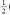(υ2 /c2). You can check for yourself, using a calculator, that this formula works pretty well for speeds that are small compared to c. Hopefully the table below will convince you if you don’t have a calculator handy. Notice that the approximate formula (which generates the numbers in the third column) is actually very accurate even for speeds as high as 10 percent of the speed of light (υ/c = 0.1), which is a usually impossible-to-reach 30 million meters per second.

After making this simplification, γmc2 is then approximately equal to mc2 +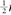2. It is at this point that we are able to realize the profoundly significant consequences of what we have been doing. For speeds that are small compared to c, we have determined that the quantity mc2 +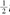2 is conserved. More precisely, it is the quantity γmc2 that is conserved, but at this stage, the former equation is much more illuminating. Why? Well, as we have already seen, the product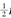2 is the kinetic energy we encountered in our example of the colliding billiard balls and it measures how much energy an object of mass m has

 v/c γ 1 +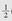(v2/c2) 0.01 1.00005 1.00005 0.1 1.00504 1.00500 0.2 1.02062 1.02000 0.5 1.15470 1.12500

TABLE 5.1

as a result of the fact that it is moving with a speed υ. We have discovered that there is a thing that is conserved that is equal to something (mc2) plus the kinetic energy. It makes sense to refer to the “something that is conserved” as the energy, but now it has two bits to it. One is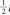2 and the other is mc2. Don’t be confused by the fact that we multiplied by c. We did that only so our final answer included the term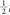2rather than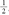2/c2, and the former is what scientists have for many generations called kinetic energy. If you like, you can christen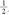2 /c2 the “kinetic mass” or any other name you care to dream up. The name is irrelevant (even if it carries the great gravitas that “energy” does). All that matters is that it is the “time component of the momentum spacetime vector,” and that is a conserved quantity. Admittedly, the equation “the time component of the momentum spacetime vector equals mc” does not have the catchy appeal of E = mc2, but the physics is the same.

Remarkably, we have demonstrated that the conservation of momentum in spacetime leads not only to a new, improved version of the conservation of momentum in three dimensions, but also to a revised law for the conservation of energy. If we imagine a system of particles all jiggling about, then we have just figured out that adding together the kinetic energy of all the particles plus the mass of all the particles multiplied by c squared we get something that is unchanging. Now, the Victorians would have been happy with the assertion that the sum of kinetic energies should be unchanging, and they would also have been happy with the assertion that the sum of the masses should be unchanging (multiplying by c squared is irrelevant when we’re thinking about what is unchanging). Our new law is consistent with that being the case, but it is much more than that. As it stands there is nothing at all preventing some of the mass from being converted into kinetic energy and vice versa, as long as the sum of these two things is always conserved. We have discovered that mass and energy are potentially interchangeable and the amount of energy we can extract from a mass m at rest (γ is equal to one in that case) is captured by the equation E = mc2.

Our friend Thales of Miletus can at last achieve complete enchantment. He rises from his bath, dripping asses’ milk onto the floor, and welcomes his concubines into his magnificent presence.

Let’s recap: We wanted to look for an object in spacetime that did the job of momentum in three-dimensional space, because momentum is a conserved quantity and therefore useful. We were able to find such an object by building it only out of things that everyone agrees upon, namely the distance in spacetime, the universal speed limit, and the mass. The spacetime momentum vector that we constructed turned out to be very interesting. By looking at the part that points along the space direction, we rediscovered the old law of momentum conservation, with a tweak for things moving close to the speed of light. But the real gold came from looking at the part of the vector that points along the time direction. This gave us an entirely new version of the law of conservation of energy. The old-fashioned kinetic energy,2, was there, but a totally new piece appeared: mc2. Thus, even if an object is standing still, it has energy associated with it, and that energy is given by Einstein’s famous equation: E = mc2.

What does it all mean? We have established that energy is an interesting quantity because it is conserved: “You can increase energy over here provided you lower it over there.” Moreover, we have established that the raw mass of an object provides a potential source of energy. We can imagine taking a blob of matter, say 1 kilogram of “stuff ” (it doesn’t matter what) and “doing something to it” so that afterward there is no 1 kilogram of stuff anymore. And by that we don’t mean the 1 kilogram has been smashed up into tiny bits, we mean that it has vanished. In fact, we can imagine an extreme scenario where all of the original mass gets used up. In its place must be 1 kilogram worth of energy (plus any energy we might have put in when we did the “doing something to it”). That energy could itself be in the form of mass, for example a few hundred grams of new “stuff ” might be created, and the remaining energy could be in the form of kinetic energy: the new stuff could be whizzing about with speed. Of course, we just made all of that up; it was an imaginary scenario. The point to appreciate is that this is the kind of thing that could be allowed by Einstein’s theory. Before Einstein, no one had dreamed that mass could be destroyed and converted into energy because mass and energy seemed to be entirely disconnected entities. After Einstein, everyone had to accept that they are different manifestations of the same type of thing. This is because we have discovered that energy, mass, and momentum must all be combined into a single spacetime object that we have been referring to as the spacetime momentum vector. Actually, its more usual name in physics circles is the energy-momentum four-vector. Just as we discovered that space and time should no longer be thought of as separate entities, so we have found that energy and momentum are shadows of a more profound object, the energy-momentum four-vector. We are fooled into thinking of them as unrelated and distinct entities because of our heavy intuitive bias to separate space and time from each other. Crucially, nature does exploit the opportunity—it is possible to convert mass into energy. If nature did not allow this to happen, then we would not even exist.

Before we unpick that rather strong statement, a further word on what we mean by “destroyed” is probably in order. We do not mean destruction in the sense that a precious vase might fall and get smashed into smithereens. After that kind of destruction you could imagine dejectedly sweeping up the pieces and weighing them—there would be no noticeable change in mass. What we mean is that the vase gets destroyed such that after the act of destruction there are fewer atoms than before and the mass is correspondingly less. This might seem like a new and controversial notion. The idea that matter is made up of tiny pieces and that we can chop the pieces up and rearrange them but never destroy them is a powerful one, dating back to Democritus in ancient Greece. Einstein’s theory overturns that view of the world and leads instead to a world in which matter is more nebulous—capable of popping into and out of existence. Indeed, that cycle of destruction and creation is today carried out routinely in the world’s particle physics accelerators. We shall come back to these matters later.

Now for the grand finale. Unfortunately, we have run out of things for Thales to do in polite company, but this is really going to be wonderful. We want to wrap up the identification of c with the speed of light. As we have been keen to stress, the important thing in the spacetime way of thinking about things is that c is a universal cosmic speed limit, not that it is the speed of light. In the last chapter we did eventually identify c as the speed of light but only after comparing to the results we found in Chapter 3. Now we can do it without resorting to ideas outside of the spacetime framework. We shall attempt to find an alternative interpretation of the c that occurs in E = mc2, other than that it is the cosmic speed limit.

The answer can be found in another bizarre and well-hidden feature of Einstein’s mass-energy equation. To investigate further, we need to step back from our approximations and write the space and time parts of the energy-momentum four-vector in their exact form. The energy of an object, which is the time part of the energy-momentum four-vector (multiplied by c), is equal to γmc2, and the momentum, which is the space part of the energy-momentum four-vector, is γmυ . Now we ask what at first sight seems to be a very weird question: What happens if an object has zero mass? A quick glance might suggest that if the mass is zero, then the object always has zero energy and zero momentum, in which case it would never influence anything and it might as well not exist. But thanks to a mathematical subtlety that is not the case. The subtlety lies in γ . Recall that γ = 1/√1—υ2/c2. If the object moves at the speed c, then the factor γ becomes infinite, because we have to take one divided by zero (the square root of zero is zero). So we have a strange situation for the very specific case in which the mass is zero and the speed is c. In the mathematical expressions for both momentum and energy, we end up with infinity multiplied by zero, which is mathematically undefined. In other words, the equations as they stand are useless but, crucially, we are not entitled to conclude that the energy and momentum are necessarily zero for massless particles. We can, however, ask what happens to the ratio of the momentum and the energy. Dividing E = γmc2 by p = γmυ leaves us with E/p = c2/υ, which for the special case υ = c leaves us with the equation E = cp, which is meaningful. Therefore, the bottom line is that both the energy and momentum could conceivably be nonzero even for an object with zero mass but only if that object travels at speed c. So Einstein’s theory allows for the possible existence of massless particles. Here is where the experiments come in handy. They have shown us that light is made up of particles called photons and that as far as anyone can tell they have zero mass. As a result, they must travel at the speed c. There is an important point here—if at some point in the future an experiment is performed that reveals that photons actually have a tiny mass, what should we do? Well, hopefully you can answer that question now. The answer is that we do nothing, except go back to Einstein’s second postulate in Chapter 3 and replace it with the statement that “the speed of massless particles is a universal constant.” Certainly c remains unchanged by the new experimental data; what changes is that we should no longer identify it with the speed at which light travels.

This is pretty profound stuff. The c in E = mc2 has something to do with light only because of the experimental fact that particles of light just happen to be massless. Historically, this was incredibly important because it allowed experimentalists like Faraday and theorists like Maxwell to gain direct access to a phenomenon that traveled at the special universal speed limit—electromagnetic waves. This played a key role in Einstein’s thinking, and perhaps without this coincidence, Einstein would not have discovered relativity. We shall never know. “Coincidence” may be the right word because, as we shall see in Chapter 7, there is no fundamental reason in particle physics that guarantees that the photon should be massless. Moreover, there is a mechanism known as the Higgs mechanism that could, in a different universe, perhaps, have given it a nonzero mass. The c in E = mc2 should therefore be seen more correctly as the speed of massless particles, which are absolutely forced to fly around the universe at this speed. From the spacetime perspective, c was introduced so we could define how to compute distances in the time direction. As such, it is ingrained into the very fabric of spacetime.

It may not have escaped your attention that the energy associated with a certain mass carries with it a factor of the speed of light squared. Since the speed of light is so great compared to everyday, run-of-the-mill speeds (the υ in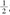2 ) it ought to come as no surprise that the energy locked away inside even quite small masses is mind-bogglingly large. We are not yet claiming to have proven that this energy can be accessed directly. But if we could get at it, then how huge an energy supply could we be, quite literally, sitting on? We can even put a number on it because we have the relevant formulas on hand. We know that the kinetic energy of a particle of mass m moving with a speed υ is approximately equal to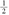2 and the energy stored up inside the mass is equal to mc2 (we shall assume that υ is small compared to c; otherwise, we would need to use the more complicated formula γmc2). Let’s play around with some numbers to get a better feel for what these equations actually mean.

A lightbulb typically radiates 100 joules of energy every second. A joule is a unit of energy named after James Joule, one of the great figures of Manchester whose intellectual drive powered the Industrial Revolution. One hundred joules every second is 100 watts, named after the Scottish engineer James Watt. The nineteenth century was a century of fantastic progress in science, now commemorated in the way we measure everyday quantities. If a city has 100,000 inhabitants, then a reasonable estimate is that it needs an electrical power supply of around 100 million watts (100 megawatts). To generate even 100 joules of energy requires a fair amount of mechanical effort. It is approximately equal to the kinetic energy of a tennis ball traveling at around 135 miles per hour, which is the service speed of a professional tennis player. You can go ahead and check this number. The mass of a tennis ball is around 57 grams (or 0.057 kilograms) and 135 miles per hour is nearly the same as 60 meters per second. If we put these numbers into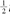2, we get a kinetic energy equal to ½ x 0.057 x 60 x 60 joules. One joule can be defined as the kinetic energy of a 2-kilogram mass traveling at 1 meter per second (that is why we converted the speed from miles per hour to meters per second), and you can do the multiplication yourself. One would therefore require a constant barrage of such tennis balls (one every second) to power just one electric lightbulb. In reality, the balls would have to travel even faster or arrive even more frequently because we would need to extract the kinetic energy from the balls, convert it to electrical energy (via a generator), and deliver it to the lightbulb. That is certainly a lot of effort to power a lightbulb.

How much mass would we need to do the same job if we could exploit Einstein’s theory and convert it all into energy? Well, the answer is that the mass should equal the energy divided by the speed of light squared: 100 joules divided by 300 million meters per second, twice. This is just over 0.000000000001 grams or, in words, one-millionth of one-millionth (i.e., one-trillionth) of 1 gram. At that rate, we need to destroy only 1 microgram of material every second to power a city. There are around 3 billion seconds in one century, so we would need only 3 kilograms of material to keep the city going for 100 years. One thing is for sure, the energy potential that is locked away within matter is on a different scale from anything we ordinarily experience, and if we could unlock it, we would have solved all of the earth’s energy problems.

Let us make one final point before we move on. The energy locked up in mass feels utterly astronomical to us here on Earth. It is tempting to say that this is because the speed of light is a very big number, but that is to emphatically miss the point. The point is rather that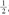2 is a very small number relative to mc2 because the velocities that we are used to dealing with are so small compared to the cosmic speed limit. The reason we live in our relatively low-energy existence is ultimately linked to the strengths of the forces of nature, particularly the relative weakness of the forces of electromagnetism and gravity. We will investigate this in more detail in Chapter 7, when we enter the world of particle physics.

It took humans around a half century after Einstein before they eventually figured out how to extract significant amounts of mass energy from matter, and the destruction of mass is exploited today by nuclear power plants. In stark contrast, nature has been exploiting E = mc2 for billions of years. In a very real sense, it is the seed of life, for without it our sun would not burn and the earth would be shrouded forever in darkness.

﻿

﻿